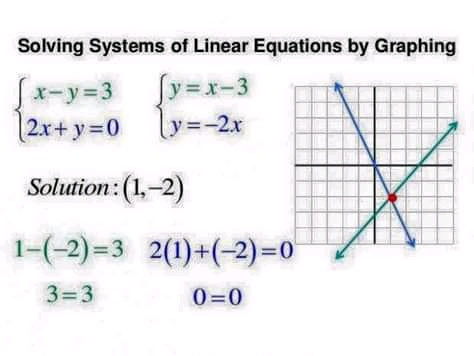Sign inScience Hightech

Science

# How to Solve Linear EquationSolving a linear Diophantine equation means that you need to find solutions for the variables x and y that are integers only. Finding integral solutions is more difficult than a standard solution and requires an ordered pattern of steps. You must first find the greatest common factor of the coefficients in the problem, and then use that result to find a solution. If you can find one integral solution to a linear equation, you can apply a simple pattern to find infinitely many more.

Setting up the Equation

Equation Step 1

1

Write the equation in standard form. A linear equation is one that has no exponents greater than 1 on any variables. To solve a linear equation in this style, you need to begin by writing it in what is called “standard form.” The standard form of a linear equation looks like {\displaystyle Ax+By=C}Ax+By=C, where {A,B}A,B and {C}C are integers.

If the equation is not already in standard form, you need to use the basic rules of algebra to rearrange or combine the terms to create the standard form. For example, if you begin with {23x+4y-7x=-3y+15}23x+4y-7x=-3y+15, you can combine similar terms to reduce the equation to {\displaystyle 16x+7y=15}16x+7y=15.

Image titled Solve a Linear Diophantine Equation Step 2

2

Reduce the equation if possible. When the equation is in standard form, check all three terms {A,B}A,B and {C}C. If there is a common factor in all three terms, then reduce the equation by dividing all terms by that factor. If you reduce evenly across all three terms, then any solution you find for the reduced equation will also be a solution for the original equation.

For example, if all three terms are even, you can at least divide by 2, as follows:

{42x+36y=48}42x+36y=48 (all terms are divisible by 2)

{21x+18y=24}21x+18y=24 (all terms now are divisible by 3)

{7x+6y=8}7x+6y=8 (this equation is as reduced as possible)

Equation Step 3

3

Check for the impossibility of a solution. In some cases, you may be able to tell immediately if there is no solution to your problem. If you see a common factor on the left side of the equation that is not shared on the right side, then there can be no solution to the problem.

For example, if both {A}A and {B}B are even, then the sum of the left side of the equation would have to be even. But if {C}C is odd, then there will be no integer solution to the problem.

{2x+4y=21}2x+4y=21 will have no integer solution.

{5x+10y=17}5x+10y=17 can have no integer solution, because the left side of the equation is divisible by 5, but the right side is not.

Thanks for reading. Click on Follow to recieve updates. Like, Comment and Share

Content created and supplied by: Patrickbaah (via Opera News )

Diophantine Equation

COMMENTS

Load app to read more comments